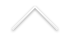É um prazer convidá-los para mais uma edição do nosso Colóquio de Geometria e Aritmética do Rio de Janeiro. As palestras serão realizadas na próxima sexta 25 de outubro, na Uff - Auditório do Bloco G - Campus Grogoatá.

#### A programação será a seguinte:

10:30: Luca Scala (UFRJ)

Título: Singularities of the Isospectral Hilbert Scheme

Resumo: The Isospectral Hilbert Scheme of Points over a surface was defined by Haiman as the blow-up of the product variety X^n of a complex algebraic surface X along the scheme-theoretic union of its pairwise diagonals; it realizes one of the parametrizations of configurations of n points over the surface X taking into account the order of the points in the configuration. It is in general a singular variety: its singularities are related on one hand to the singularities of the boundary of the (standard) Hilbert scheme of points and on the other hand to those of the union of the pairwise diagonals in the product X^n: the latter, in case of the affine plane, coincide with a subspace arrangement. Haiman himself showed that the Isospectral Hilbert scheme is normal and Gorenstein, but lots of questions about the singularities of this variety remain unanswered; we state three conjectures on this point. We prove that the singularities of B^n are canonical if n ≤5, log-canonical if 6 ≤n≤7 and that they are definitely not log-canonical if n≥9. We also give two explicit resolutions of B^3, one crepant and one S_3-equivariant.

11:30: café.

12:00: André Contiero (UFMG)

Título: On the strata in the moduli of pointed curves given by Weierstrass gaps

Resumo: We will present a new lower bound for the dimension of the moduli space of pointed curves with prescribed Weierstrass semigroup at the marked point. This new lower bound is derived from Pinkham's equivariant deformation theory and improves all the previous ones that we are aware. Few results of a working in progress on the rationality of these moduli spaces will also be presented.

As palestras do Colóquio de Geometria e Aritmética (COLGA) do Rio de Janeiro serão realizadas em 30 de agosto (sexta-feira), no Instituto de Matemática, UFRJ - CT sala C116 – Ilha do Fundão.

Programação:

09:30 às 10:30: Applications of curves over finite fields to polynomial problems, Daniele Bartoli (Università degli Studi di Perugia - Itália).

Resumo: Algebraic curves over finite fields are not only interesting objects from a theoretical point of view, but they also have deep connections with different areas of mathematics and combinatorics. In fact, they are important tools when dealing with, for instance, permutation polynomials, APN functions, planar functions, exceptional polynomials, scattered polynomials, Moore-like matrices. In this talk I will present some applications of algebraic curves to the above mentioned objects.

10:30 às 11:00: Pausa para o café.

11:00 às 12:00: Inflection of linear series on hyperelliptic curves over arbitrary fields (joint with I. Biswas, I. Darago, C. Han and C. Garay López), Ethan Cotterill (UFF).

Resumo: According to Plucker's formula, the total inflection of a linear series (L,V) on a complex algebraic curve C is fixed by numerical data, namely the degree of L and the dimension of V. The problem of describing the k-rational inflectionary locus of a Gal(k-bar/k)-linear series (L,V) when k is a non-algebraically closed field is significantly more subtle. For example, the topology of the real inflectionary locus of a real linear series depends in a nontrivial way on the topology of the real locus of C. I will describe joint work with Biswas and Garay López in which we study this dependency when C is hyperelliptic and (L,V) is a complete series. Our main tool is a nonarchimedean degeneration, which allows us to relate the (real) inflection of complete series to the (real) inflection of incomplete series on elliptic curves. I will also describe work in progress with Darago and Han in which we compute k-rational inflectionary loci valued in the Grothendieck--Witt group GW of an arbitrary field k. To do so, we apply the A^1 homotopy theory of Morel, Voevodsky, Levine, Kass and Wickelgren.

As palestras serão realizadas na próxima sexta-feira, 28 de Junho, na UFRJ - Instituto de Matemática, UFRJ - CT, Sala C208 – Ilha do Fundão.

Programação:

09:30 às 10:30: The Hurwitz curve over a finite fielde and its Weierstrass points for the morphism of lines, Herivelto Borges (USP - São Carlos).

Resumo: Let$\mathcal{X}$ be an irreducible algebraic curve defined over an algebraically closed  field$\mathbb{K}$   of characteristc$p\geq 0$. The genus of$\mathcal{X}$  is certainly the most famous  birational invariant of$\mathcal{X}$. If$\mathbb{K}(\mathcal{X})$ denotes the function field  of$\mathcal{X}$, the group all$\mathbb{K}$-automorphisms  of$\mathbb{K}(\mathcal{X})$ is called  {\it automorphism group} of$\mathcal{X}$, and it is denoted by Aut$(\mathcal{X})$. Such group is another birational invariant of$\mathcal{X}$, and the study of Aut$(\mathcal{X})$ has  become a central problem within the theory of algebraic curves. In this talk, we will consider smooth Hurwitz curves$\mathcal{H}_n: \, XY^n+YZ^n+X^nZ=0,$

over the finite field$\mathbb{F}_{p}$ and  provide  an  explict  description of  its Weierstrass points for the  morphism of lines. That is, we will completely charaterize the special set  of points$P\in \mathcal{H}_n$ for which the intersection multiplicity$I(P,\mathcal{H}_n \cap T_{P}\mathcal{H}_n)$ is somewhat large. As a consequence, the full automorphism  group Aut$(\mathcal{H}_n)$, as well as the genera of all Galois subcovers  of$\mathcal{H}_n$ will be presented. In addition, we will discuss how this information can be used to bound the number of$\mathbb{F}_p$-rational points  on$\mathcal{H}_n$ via St\"ohr-Voloch Theory.

10:30 às 11:00: Pausa para o café.

11:00 às 12:00: Componentes tipo pullback do espaço de folhações de codimensão um em$\mathbb{P}^n$, Viviana Ferrer (UFF - Niterói).

Resumo: O espaço de folheações holomorfas  de codimensão um e grau d em$\mathbb{P}^n$ tem uma  componente irredutível cujo elemento genérico pode ser escrito como o pullback$F^*\mathcal{F}$onde$\mathcal{F}$ é uma folheação genérica de$\mathbb{P}^2$  e$F :\mathbb{P}^n\dasharrow \mathbb{P}^2$ é um mapa racional. (Cerveau, Lins-Neto, Edixhoven, 2001). Nesta palestra mostraremos como são encontradas fórmulas (polinômios em d) para o grau desta componente no caso de pullback linear.Mostraremos também quais são as dificuldades para calcular o grau de componentes dadas por pullback de folheações por mapas não lineares.